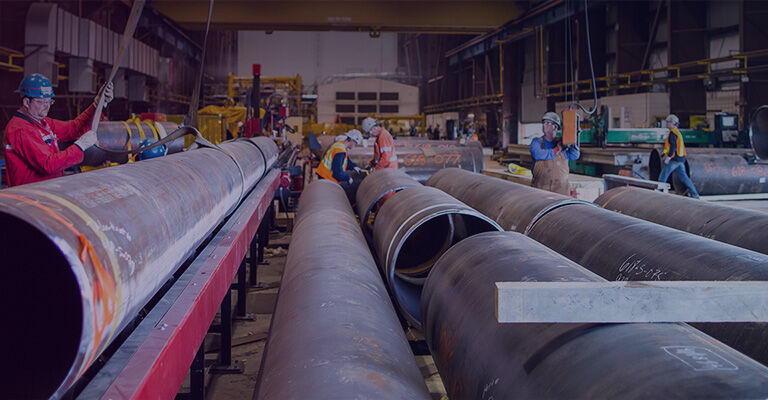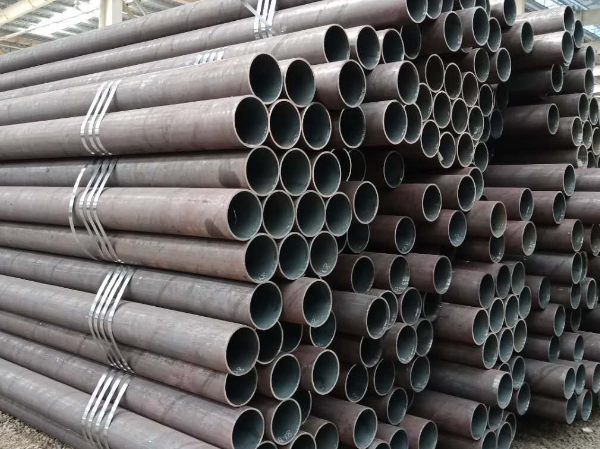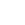## Company News## Company NewsYou are in :  Home  >  News  >  Company News

# Seamless steel pipe weight calculation

Date:2021-11-04View:1435Tags:smls pipe, seamless pipe weight calculation
Seamless steel pipe weight calculation formula:

The weight of seamless steel pipe per meter W=0.02466*S(D-S).

Symbol meaning: D=outer diameter, S=wall thickness.

For example: a seamless steel pipe with an outer diameter of 60mm and a wall thickness of 4mm, find the weight per meter.
Solution: Weight per meter=0.02466*4*(60-4)=5.52Kg.

The calculation formula of seamless steel pipe theoretical weight seamless steel pipe W=0.0246615(D-S)*SW.Extended information:
1. The formula for calculating the weight of carbon steel pipes with square and rectangular (rectangular) cross-sections:

The unit of weight per meter: kg/m (kilogram/meter) & lb/ft (lb/ft).
Calculation formula: kg/m = (OC-4WT) * Wt * 0.00785
Among them: OC is the outer circumference, WT is the wall thickness; square: OC=4*a, rectangle: OC=2a+2b. a,b are side lengths.

2. The formula for calculating the weight of a circular cross-section carbon steel pipe:

Calculation formula: kg/m = (OD-WT) * WT * 0.0246615.

Among them: OD is the outer diameter and WT is the wall thickness.